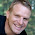## I don't post every day, so the best way to not miss anything is to subscribe by email

### What's the difference between simple and elementary regions?

Simple regions are a topic that comes up in vector calculus.

First, let's understand the difference between x-simple and y-simple regions. Visually, the Type 1 region seen here is an example of a y-simple region and the Type 2 region is an example of an x-simple region. See any textbook on vector calculus for a formal definition.
But intuitively, a y-simple region is any region of space that can be bounded between two functions y=g1(x) and y=g2(x). If the region requires more than two such functions to bound it, then it’s not y-simple. The same idea applies to x-simple regions, but the two functions are x=g1(y) and x=g2(y).

The idea, really, is a region is simple if you only need two functions to define its boundary.
Now, here's the easy part.

A region is said to be “simple” if it is both y-simple and x-simple.

A region is said to be “elementary” if it is y-simple or x-simple.

Bonus idea: Do you see why this is useful for integration? Integration is defined from point A to point B. If a region is simple it means you can write limits of integration easily; the functions g1 and g2 are the limits of integration.

Source of image used

#### 1 comment:

1.Thanks for information!